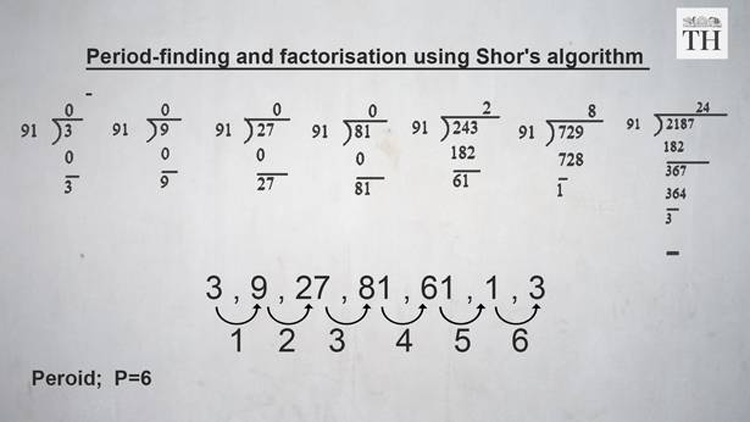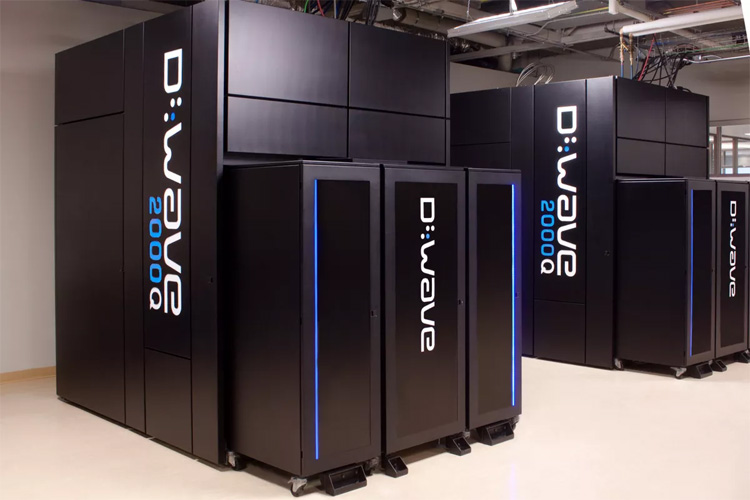We have trusted Internet banking and mobile wallet apps with our personal and financial information, often based on assurances about their “strong encryptions” that current computers would take millions of years to crack using brute force methods.

But what if computing power rose on a mind-boggling scale in a short period of time?Google's Bristlecone quantum computing chip.

The zeal with which researchers are developing quantum computers could change cryptography as we know it. Last month, Google unveiled a 72-qubit gate-based superconducting system that it claims “will be able to outperform a classical supercomputer on a well-defined computer science problem, an achievement known as quantum supremacy.”

Theoretically, quantum computers can successfully sift through the near-infinite combinations of public key cryptography, a system which uses two keys for decryption. Data encryption algorithms, such as RSA (cryptosystem) that is used in payment gateways, have been broken by tests involving Shor’s algorithm running on quantum computers.

Here is all you need to know about the technology that could potentially force a rethink of governing standards in information security:

### What is quantum computing?

Semiconductor-based processors for conventional computers encode data into binary digits, or bits, which can be in either of two states -- one and zero.

A quantum bit or qubit -- the building block of quantum computers -- can encode one and zero, and also a number of other states simultaneously through superimposition and entanglement, as explained by quantum mechanics.

Quantum mechanics shows how particles and waves have a dual nature. Particles like electrons tend to behave like waves, whereas light waves also display particle nature.Bloch sphere representation of a qubit.
A Bloch sphere is the logical representation of a qubit. The two poles of the sphere represent the states one and zero. At any given time, a qubit can also exist in a superimposed quantum state, which can be represented as one among the near-infinite points on the sphere.

To better understand this, consider the case of a coin flipped in the air. When airborne, the coin can be thought of as being a combination of heads and tails simultaneously. Quantum computers make use of not just the heads or tails position to encode data, but also the combination states in between, exacted using probability.

### So how can it crack encryption?

The cryptographic standards of today exploit the inadequacy of classical computers to work backwards to find the factors of extremely large numbers. Quantum computers outsmart their classical peers by parsing the range of possibilities into well-defined periods and using quantum states to check for the answer in different periods simultaneously.

Modern computers evolved from primitive counting machines, and to this day, binary addition remains the foundation of all other operations in a computer’s repertoire. The act of multiplying two numbers, whatever their size is a feat that most conventional computers can accomplish without breaking a sweat. However, things get more complicated if computers are asked to calculate the factors of a very large number, say, one which comprises of a few hundred digits. Such tasks are near-impossible for most computers and even sophisticated workstations with greater processing capability.

This vulnerability is taken advantage of by cryptographic algorithms like RSA. Whenever you send a message on WhatsApp, buy a book on Amazon, or order food on Zomato, the RSA algorithm is called into action and encrypts your personal details inside a long stream of digits.

The website or entity, with which you want to transact, generates a public key, which is an extremely large number. This is publicly available and contains your financial credentials such as credit card number and bank details.

The key to this is the product of two prime numbers (N = p * q) which is known only to the seller. In order to obtain the key, and hence hijack your account, hackers have to factor the large public key generated by the seller. The numerical heft of the number makes it computationally impossible for the system to be breached. However, quantum computers running Shor’s algorithm could alter the status quo.

### Shor’s algorithm

The eponymous algorithm for integer factorization was formulated by Peter Shor in 1994. The solution proposed by Shor in finding the prime factors of an integer has subsequently found application in quantum computing. Peter Shor was awarded the Gödel Prize for his efforts.

Shor’s algorithm consists of two parts, the first of which is a period finding problem that can be solved on a regular computer. The second part, which is responsible for expediting the speed of computation, involves finding the period using the quantum Fourier transform. Here is a brief overview of the algorithm.

Step 1: Let m be a random non-zero integer. Use the greatest common divisor (gcd) algorithm for classical computing to find the gcd (N,m).

[gcd is the largest positive integer that divides each of the integers. For example, the gcd of 30 and 12 is 6.]

If, gcd (N,m) = 1, proceed to step 2. Otherwise, a non-trivial solution has been found.

Step 2: Find the period P of: m mod N, m2 mod N, m3 mod N, m4 mod N, …

[The modulo operation finds the remainder after one number is divided by another (sometimes called modulus). Given two positive numbers, m and N, m modulo N (abbreviated as m mod N) returns the remainder of the division of m by N.]

Step 3: If the period P is odd, go back to step 1 and proceed with another random integer. If P is even, go to the next step.

Step 4: Check if: mP/2  + 1 is not equal to 0 mod N. If true, proceed to the next step, otherwise, go to step 1.

Step 5: Find the gcd (mP/2 -1, N).

The answer is a non-trivial prime factor of N. More importantly, this gives us the key to breaking the RSA cryptosystem.

The algorithm might seem confusing, but it isn’t. Let us try and solve Shor’s algorithm by taking N=91.

Step 1: Let us consider a random number 3 such that N-91, m=3

gcd (3,91) = 1  [since they have no common factors]

Therefore, proceed to the next step.

Step 2: Find the period P of : 3 mod 91, 32 mod 91, 33 mod 91, 34 mod 91, …

A quantum computer finds the period of the above function by performing a quantum Fourier transform. This relies on the ability of quantum computers to be in multiple states at the same time, thereby allowing the function to be evaluated simultaneously for different values.In this case, we manually find the period P through repeated long division. When remainder obtained by the modulo function starts repeating, we have found the period of the function.

Here, the remainder returns to 3 after 6 rounds. Hence, P=6.

Step 3: Since P = even, we proceed to the next step.

Step 4: We check if: mP/2  + 1 is not equal to 0 mod N

Here, 3P/2 + 1 = 36/2 + 1 = 33 + 1 =27 + 1 = 28, which is not equal to 0 mod N

Step 5: Find a factor q such that q = gcd (mP/2 -1, N)

Here, q = gcd (3P/2 – 1, N) = gcd (36/2 – 1, 91) = gcd (26, 91) = 13

Since 26 = 13 *2

91 = 13 *7

Hence p = 13 , q = 7. RSA has been broken for this example.

However, in real life N will be much larger than 91, making factorisation impossible for classical computers, leave alone solving the period-finding problem by long division. If at all human beings were to manually factorise a 1000-digit number, it would take multiple generations of a family, billions of reams of paper, and an equal number of pencil stubs, to obtain the required factors.

While the intractability of modern cryptography is a cause of great civilisational triumph, the dawn of quantum supremacy could be the undoing of society since almost all facets of life, from communication to commerce, are now conducted online.A D-Wave quantum computer at Quantum Artificial Intelligence Laboratory (QuAIL).

However, we may need to start worrying just yet. Although Google, IBM, and D-Wave Systems have made giant strides in increasing the number of qubits in a quantum processor, mass production still remains a pipe dream.

### Post-quantum cryptography

Dystopian fantasies cannot get any bleaker than an army of hackers equipped with quantum computers. The prohibitive cost of developing these machines has insofar kept them away from the reach of miscreants. However, information security wonks are taking no chances.

The best antidote against quantum computers has been found to be cryptosystems that employ the same principles derived from physical phenomena as its nemesis– namely the duality of particles and waves, and the existence of quantum states.

Post-quantum cryptography has been focused on six main approaches of which lattice and multivariate-based cryptosystems are apart. These are said to be quantum-resistant, but the cost overheads and time latency involved in authentication make them unsuitable in dealing with heavy traffic in real-world situations.

by Rohan Abraham
via The Hindu